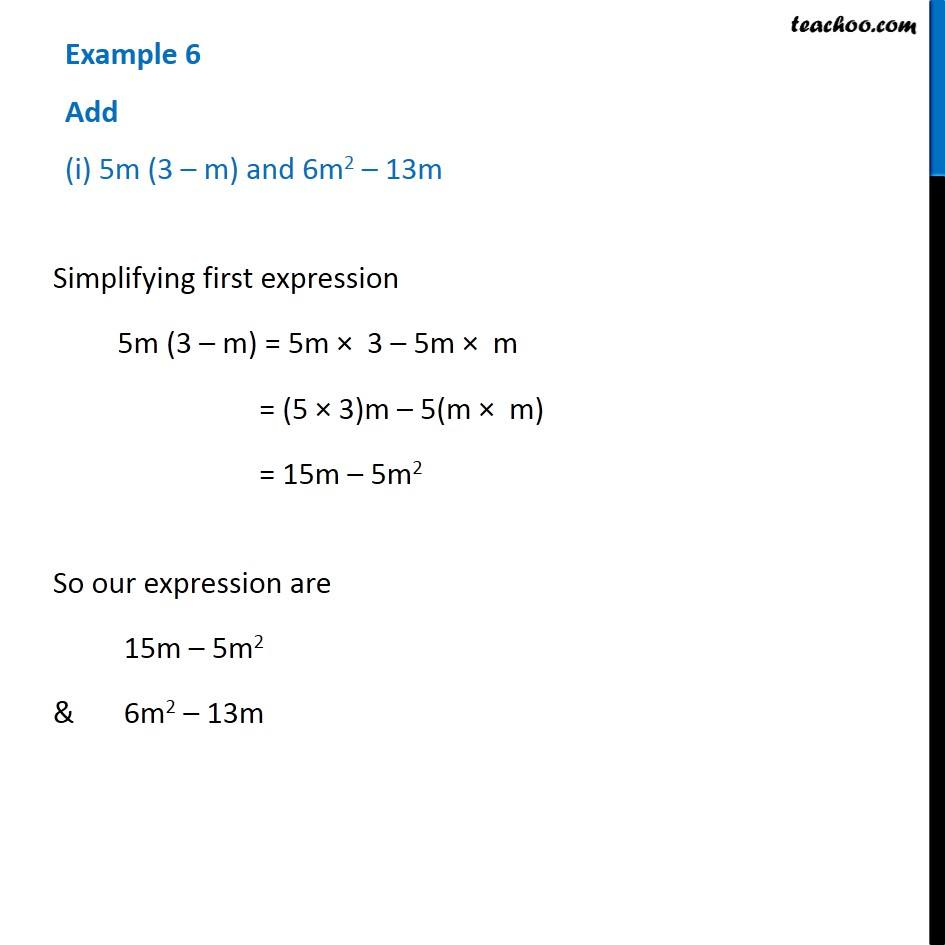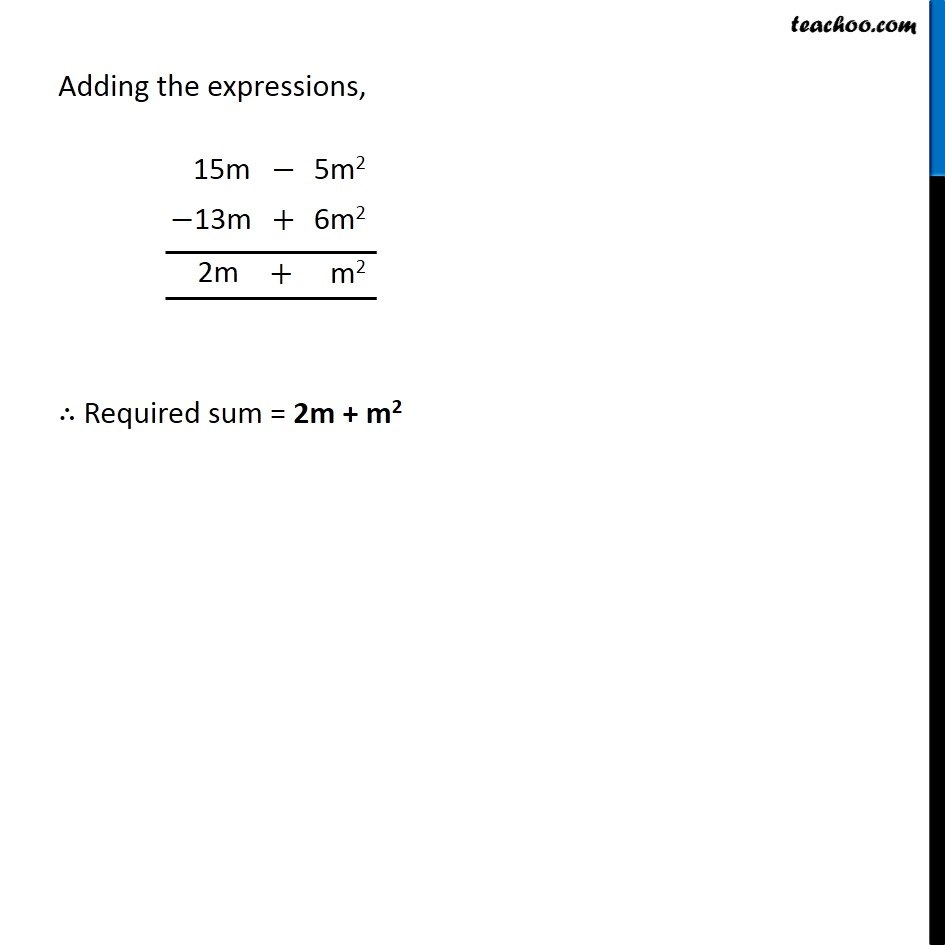Multiplication of Monomials by Binomials and Trinomials

Chapter 8 Class 8 Algebraic Expressions and Identities
Concept wiseLearn in your speed, with individual attention - Teachoo Maths 1-on-1 Class

### Transcript

Example 6 Add (i) 5m (3 – m) and 6m2 – 13m Simplifying first expression 5m (3 – m) = 5m × 3 – 5m × m = (5 × 3)m – 5(m × m) = 15m – 5m2 So our expression are 15m – 5m2 & 6m2 – 13m Adding the expressions, ∴ Required sum = 2m + m2